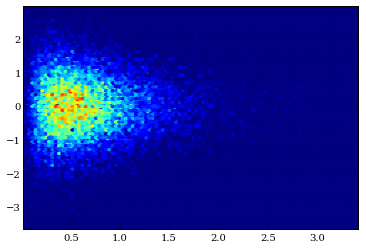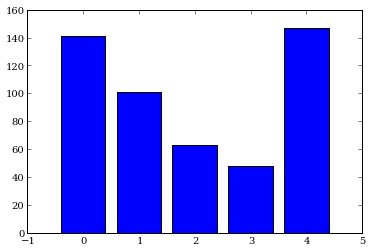# CythonGSL Demo¶

CythonGSL provides a Cython interface for the GNU Scientific Library (GSL).

Cython is the ideal tool to speed up numerical computations by converting typed Python code to C and generating Python wrappers so that these compiled functions can be called from Python. Scientific programming often requires use of various numerical routines (e.g. numerical integration, optimization). While SciPy provides many of those tools, there is an overhead associated with using these functions within your Cython code. CythonGSL allows you to shave off that last layer by providing Cython declarations for the GSL which allow you to use this high-quality library from within Cython without any Python overhead.

In :
%load_ext cythonmagic
from matplotlib import pyplot as plt

In :
%%cython -lgsl -lgslcblas
'''
Gibbs sampler for function:

f(x,y) = x x^2 \exp(-xy^2 - y^2 + 2y - 4x)

using conditional distributions:

x|y \sim Gamma(3, y^2 +4)
y|x \sim Normal(\frac{1}{1+x}, \frac{1}{2(1+x)})

Original version written by Flavio Coelho.
Tweaked by Chris Fonnesbeck.
Ported to CythonGSL Thomas V. Wiecki.
'''
cimport cython
from cython_gsl cimport *

import numpy as np
cimport numpy as np

from libc.math cimport sqrt

cdef gsl_rng *r = gsl_rng_alloc(gsl_rng_mt19937)

@cython.boundscheck(False)
@cython.wraparound(False)
@cython.cdivision(True)
def gibbs(int N=20000, int thin=500):
cdef:
double x = 0
double y = 0
Py_ssize_t i, j
np.ndarray[np.float64_t, ndim=2] samples = np.empty((N, 2), dtype=np.float64)

for i in range(N):
for j in range(thin):
x = gsl_ran_gamma(r, 3, 1.0 / (y * y + 4))
y = gsl_ran_gaussian(r, 1.0 / sqrt(x + 1))
samples[i, 0] = x
samples[i, 1] = y
return samples

In :
posterior = gibbs()

In :
plt.hexbin(posterior[:, 0], posterior[:, 1])

Out:
<matplotlib.collections.PolyCollection at 0xb9c554c>In :
%%cython -l gsl -l gslcblas

cimport cython
from cython_gsl cimport *

import numpy as np
from numpy cimport *

cdef gsl_rng *r = gsl_rng_alloc(gsl_rng_mt19937)

def multinomial(ndarray[double, ndim=1] p, unsigned int N):
cdef:
size_t K = p.shape
ndarray[uint32_t, ndim=1] n = np.empty_like(p, dtype='uint32')

# void gsl_ran_multinomial (const gsl_rng * r, size_t K, unsigned int N, const double p[], unsigned int n[])
gsl_ran_multinomial(r, K, N, <double*> p.data, <unsigned int *> n.data)

return n

In :
sample = multinomial(np.array([.3, .2, .1, .1, .3], dtype='double'), 500)

In :
plt.bar(range(5), sample, align='center')

Out:
<Container object of 5 artists># Speed comparison¶

Pure Python version:

In :
import random, math, numpy

def gibbs_python(N=20000, thin=500):
x = 0
y = 0
samples = np.empty((N, 2))

for i in range(N):
for j in range(thin):
x = random.gammavariate(3, 1.0 / (y * y + 4))
y = random.gauss(1.0/(x+1),1.0 / math.sqrt(x + 1))
samples[i, 0] = x
samples[j, 1] = y
return samples

In :
%timeit gibbs_python()

1 loops, best of 3: 56.9 s per loop

In :
%timeit gibbs()

1 loops, best of 3: 2.48 s per loop